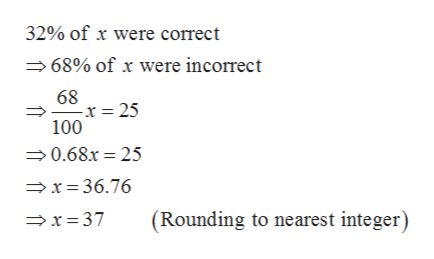# A student missed 25problems on a biology test and received a grade of 32%. If all the problems were of equal value, how many problems were on the test? Follow the problem-solving process and round your answer to the nearest integer.Answer

Question
3 views

A student missed 25problems on a biology test and received a grade of 32%.
If all the problems were of equal value, how many problems were on the test? Follow the problem-solving process and round your answer to the nearest integer.

check_circle

Step 1

Given,

Let x be the number of problems in the biology test.

Step 2

Therefore,&n...help_outlineImage Transcriptionclose32% of x were correct 68% of x were incorrect 68 -x= 25 100 0.68.r 25 x=36.76 (Rounding to nearest integer) x=37 fullscreen

### Want to see the full answer?

See Solution

#### Want to see this answer and more?

Solutions are written by subject experts who are available 24/7. Questions are typically answered within 1 hour.*

See Solution
*Response times may vary by subject and question.
Tagged in

### Equations and In-equations